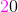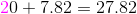# AP Chemistry : Significant Figures

## Example Questions

← Previous 1

### Example Question #1 : Significant Figures

How many significant figures should the answer to this calculation contain?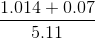Explanation:

The number of significant figures in the final answer will ultimately be determined by the term in the equation that has the fewest significant figures. Let us examine the components of the expression we are given.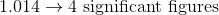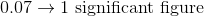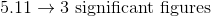Since the least precise term in the expression has only one significant figure, our final answer will have only one significant figure.

### Example Question #2 : Significant Figures

Using a graduated cylinder, you measure out 11 mL of water. You then go to an analytical balance and find the water to weigh 11.9273g.

What is the measured density of the water to the correct number of significant figures?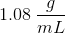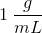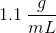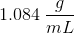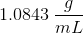Explanation: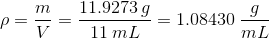When dividing two numbers, the answer can only have as many significant figures as the number with the fewest significant figures. The mass has 6 significant figures while the volume only has 2, therefore the answer can only have 2. Don't forget to round!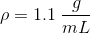### Example Question #3 : Significant Figures

How many significant figures are in the number 0.00150?Explanation:

Remember that all leading zeroes are not significant figures.

So for 0.00150, we start counting significant figures where the 1 is. The last 0 is a significant figure because it is a trailing zero found after the decimal point.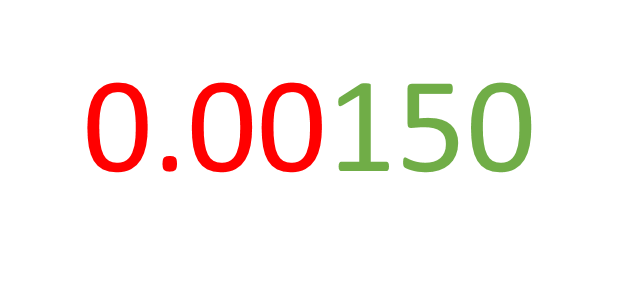The digits in red are not significant. The digits in green are significant.

### Example Question #4 : Significant Figures

How many significant figures are in the number 0.50210?Explanation: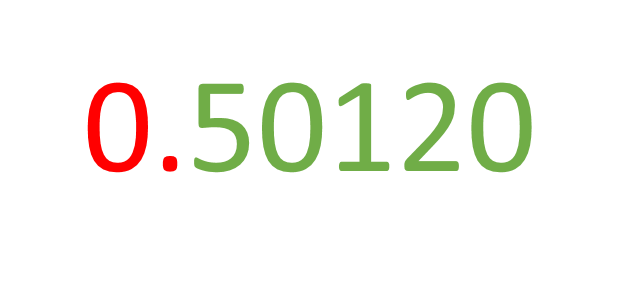The digit in red is not significant because this is a leading zero. All the digits in green are significant. The first 0 is significant because it is sandwiched between two non-zero digits. The last 0 is also significant because it is a trailing zero that comes after a decimal point. Thus, this has 5 significant figures.

### Example Question #5 : Significant Figures

Calculate and give the answer using the correct number of significant figures.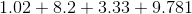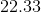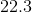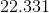Explanation: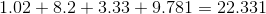Because this is addition, the result of the calculation needs to have the same number of decimal places as the number with the fewest decimal places. Since 8.2 has the least number of places after the decimal point, the answer must also only have 1 digit after the decimal point.

### Example Question #6 : Significant Figures

Calculate and give the answer using the correct number of significant figures.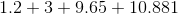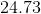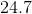Explanation:

First, do the calculation.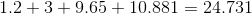Because this is addition, the result of the calculation needs to have the same number of decimal places as the number with the fewest decimal places. Since 3 has the least number of places after the decimal point, the answer must not have any decimal points. The answer will round up to 25.

### Example Question #7 : Significant Figures

Calculate and give the answer with the correct number of significant figures.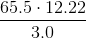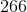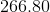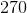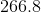Explanation:

First, do the calculation.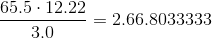Recall that for multiplication and division, the answer uses the least number of significant figures found in the question. In this case, 3.0 has the least number of significant figures at 2. The final answer should then be rounded up to only have 2 significant figures also. Thus we round up to 270.

### Example Question #8 : Significant Figures

Calculate and give the answer using the correct number of significant figures.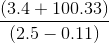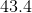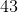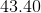Explanation:

For multistep calculations, make sure to keep track of the number of significant figures at the end of each step so we know how many significant figures to round to at the end of the entire calculation. To maintain accuracy, do not round intermediate steps. Do the addition and subtraction in the parentheses first.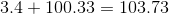Recall that for addition, the result needs to have the same number of digits after the decimal point as the number with the fewest decimal points in the question. Our answer from the addition should then only have 4 significant figures. To remember that the answer should only have 4 significant figures, the last significant digit will be highlighted: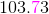Next, do the subtraction.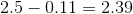Since the rules for significant figures for addition and subtraction are the same, our answer here should only have 2 significant figures. The last significant figure from this step will also be highlighted: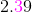Now, divide these two numbers: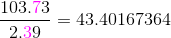Now, this is when the highlighting of significant digits in earlier steps becomes important. Recall that for multiplication and division, the result has the same number of significant figures as the factor with the least number of significant figures.

Round the final answer to 2 significant figures to reflect the least amount of significant figures found in the division. Thus 43.40167364 rounds down to 43.

### Example Question #9 : Significant Figures

Calculate and give the answer using the correct number of significant figures.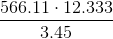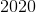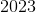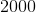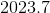Explanation:

First, carry out the calculation.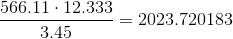Recall that for multiplication and division, the answer uses the least number of significant figures found in the question. In this case, 3.45 has the least number of significant figures at 3. The final answer should then be rounded up to only have 3 significant figures. 2023.720183 rounded to three significant figures is 2020.

### Example Question #10 : Significant Figures

Calculate and give the answer using the correct number of significant figures.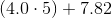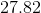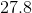Explanation:

For multistep calculations, make sure to keep track of the number of significant figures at the end of each step so we know how many significant figures to round to at the end of the entire calculation. To maintain accuracy, do not round intermediate steps. First, calculate what's found inside the parentheses.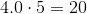Recall that for multiplication and division, the answer uses the least number of significant figures found in the question. In this case, 5 has the least number of significant figures at 1. The final answer should then be rounded up to only have 1 significant figure. The last significant digit will be highlighted: